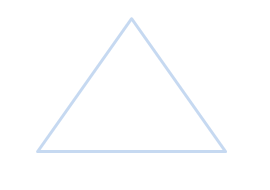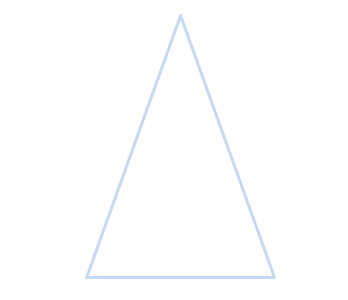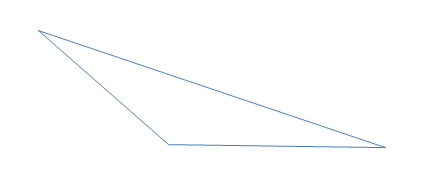## Types of Triangles

Though we've been introduced to the concept of 'triangles' since kindergarten, what we may not know is that there are so many different types of triangles. That's what we will be learning in this lesson!

Remember that there are 6 types of triangles:
• Equilateral Triangle
• Isosceles Triangle
• Scalene Triangle
• Right Triangle
• Obtuse Triangle
• Acute Triangle

## ExamplesEQUILATERAL TRIANGLEISOSCELES TRIANGLE

##SCALENE TRIANGLERIGHT TRIANGLEOBTUSE TRIANGLEACUTE TRIANGLE